# HBSE 9th Class Maths Solutions Chapter 5 Introduction to Euclid’s Geometry Ex 5.1

Haryana State Board HBSE 9th Class Maths Solutions Chapter 5 Introduction to Euclid’s Geometry Ex 5.1 Textbook Exercise Questions and Answers.

## Haryana Board 9th Class Maths Solutions Chapter 5 Introduction to Euclid’s Geometry Exercise 5.1

Question 1.
Which of the following statements are true and which are false ? Give reasons for your answers:
(i) Only one line can pass through a single point.
(ii) There are an infinite number of lines which pass through two distinct points.
(iii) A terminated line can be produced indefinitely on both the sides.
(iv) If two circles are equal, then their radii are equal.
(v) In figure 5-24, if AB = PQ and PQ = XY, then AB = XY.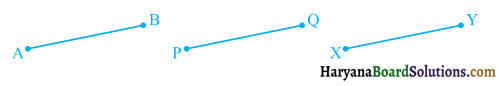Solution :
(i) False, because an infinite number of lines can be drawn passing through a given point see in the figure.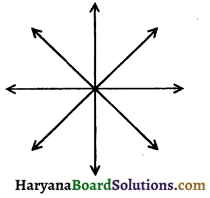(ii) False, because by Euclid’s postulate 1, a straight line may drawn from any one point to any other point.
(iii) True, because by Euclid’s postulate 2, a terminated line can be produced indefinitely on both the sides.
(iv) True, because, if you superimpose the region bounded by one circle on the other, then they coincide, so their centres and boundaries coincide. Therefore, their radii will be equal.
(v) True, because by Axiom 1, things which are equal to the same things are equal to one another.Question 2.
Give definition for each of the following terms. Are there other terms that need to be defined first? What are they, and how might you define them?
(i) Parallel lines
(ii) Perpendicular lines
(iii) Line segment
(v) Square.
Solution:
(i) Parallel lines: The straight lines, which lie in the same plane and do not meet at any point on producing on either side, are called parallel lines. In the given figure, lines I and m are parallel lines. We write l || m.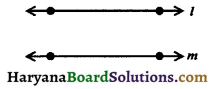(ii) Perpendicular lines : If two lines AB and CD intersect at right angle, then they are known as perpendicular lines. We write AB ⊥ CD.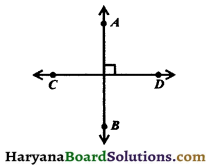(iii) Line segment: Given two distinct points A and B on the line l, the connected part (segment) of the line with endpoints at A and B, is called the line segment AB.
Line segment AB is denoted by the symbol $$\overline{A B}$$.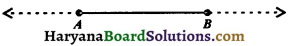(iv) Radius of a circle: The distance from the centre to any point on the circle is called the radius of the circle. In the figure OA and OB are same radii of the circle.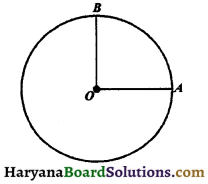(v) Square of a circle : A plane figure bounded by four straight lines having all its four interior angles right angles and all its sides of equal length. It’s diagonals bisect one another at right angles.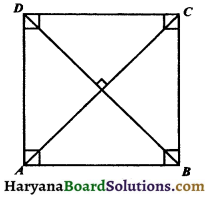In the figure, ABCD is a square.
Yes, the other terms that need to be defined first are :
(i) point,
(ii) plane,
(iii) line,
(iv) ray,
(v) angle,
(vi) circle,

(i) Point: A point is mark of position, which has no length, no breadth and no thickness. We represent a point by capital lellers A, B, P, Q etc. The given figure shows the point A.(ii) Plane: A plane is a surface such that every point of the line joining any two points on it, lies on it. The surface of the top of the table, surface of the smooth blackboard etc. are the close examples of a plane. Surfaces are limited in extent but geometrical plane extends endlessly in all directions.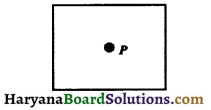(iii) Line: A line has length, but no breadth or thickness. A line has no ends points. A line has no definite length. It can be extended infinitely in both the directions. The given figure shows the line $$\overleftrightarrow{A B}$$.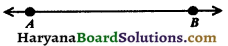(iv) Ray: It is a straight line which starts from a fixed point and moves in the same direction.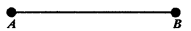A ray has one endpoint and it has no definite length. The given figure shows the ray $$\overrightarrow{A B}$$

(v) Angle: An angle is formed when two line segments or two rays have a common end point. The angle formed by the two rays AB and AC is denoted by the symbol ∠BAC or ∠CAB or simply ∠A. AB and AC are the arms of the angle and their common point A is the vertex.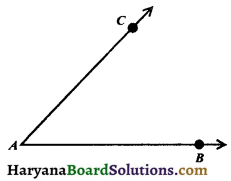(vi) Circle: A plane curve consisting of all points at a given distance from a fixed point is known as circle.
The fixed point is called the centre (figure 5.36) of the circle.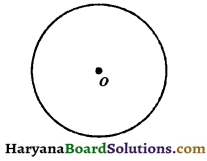(vii) Quadrilateral: A closed figure bounded by four line segments is called a quadrilateral.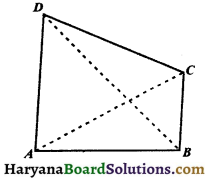The given figure shows a quadrilateral ABCD. It has four line segments AB, BC, CD and DA. The points A, B, C and D are its four vertices. It has four angles namely ∠A, ∠B, ∠C and D. AC and BD are called it’s diagonals.

Question 3.
Consider two postulates given below:
(i) Given any two distinct points A and B, there exists a third point which is in between A and B.
(ii) There exist at least three points that are not on the same line.
Do these postulates contain any undefined terms? Are these postulates consistent? Do they follow from Euclid’s postulates? Explain.
Solution:
There are several undefined terms which the students should list. They are consistent because they deal with two different situations.
(i) Says that the given two points A and B, there is a point lying on the line in between them.
(ii) Says that given A and B, you can take C not lying on the line through A and B.
These ‘postulates’ do not follow from Euclid’s postulates. However, they follow from axiom 5 1 given two distinct points, there is a unique line that passes through them.Question 4.
If a point C lies between two points A and B such that AC = BC, then prove that AC = $$\frac {1}{2}$$AB. Explain by drawing the figure.
Solution:
We have a point C lying between A and B, such that
AC = BC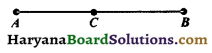∴ AC + CB = AB, (By axiom 4)
But AC = BC
∴ AC + AC = AB
⇒ 2AC = AB
⇒ AC = $$\frac {1}{2}$$AB. Hence proved

Question 5.
In question 4, point C is called a midpoint of line segment AB. Prove that every line segment has one and only one mid-point.
Solution :
Let D be another mid-point of AB.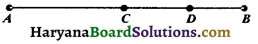But C is the midpoint of AB, (given)
∴ AC = CB
Subtracting (ii) from (i), we get
AD – AC = BD – CB ………..(ii)
⇒ CD = – CD
⇒ CD + CD = 0
⇒ 2CD = 0
⇒ CD = 0
∴ C and D coincide.
Hence, every line segment has one and only one midpoint.
ProvedQuestion 6.
In figure 5.40, if AC = BD, then prove that AB = CDSolution:
We have, AC = BD ………..(i)
Since, point B lies between A and C.
Therefore, AC = AB + BC ………..(ii)
and point C lies between B and D.
∴ BD = BC + CD … (iii)
From (i), (ii) and (iii), we get
AB + BC = BC + CD
⇒ AB + BC – BC = BC + CD – BC, (subtracting BC from both sides)
⇒ AB = CD. Hence proved

Question 7.
Why is Axiom 5, in the list of Euclid’s axioms, considered a ‘universal truth’ ? (Note that the question is not about the fifth postulate).
Solution:
Axiom 5, in the list of Euclid’s axiom’s is true for anything in any part of the world, this is a universal truth.